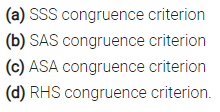# Which congruence criterion do you use in the following?

Which congruence criterion do you use in the following?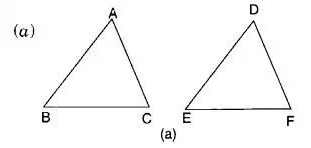Given:
So.
AC = DF
AB = DE
BC = EF
so ∆ ABC = ∆ DEF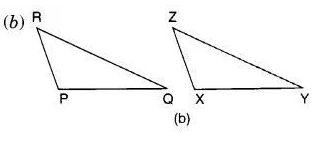Given: ZX = RP
RQ = ZY
∠PRQ = ∠XZY
So, ∆ PQR ≅ ∆ XYZ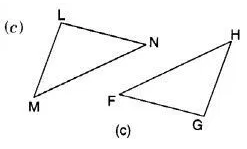Given : ∠MLN = ∠ FGH
∠NML = ∠GFH
ML = GF
So, ∆ LMN ≅ ∆ GFH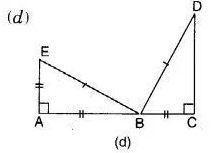Given : EB = DB
AE = BC
∠A = ∠C = 90°
So, ∆ ABE ≅ ∆ CDB